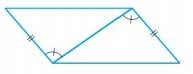Chapter A, Problem 4E### Algebra and Trigonometry (MindTap ...

4th Edition
James Stewart + 2 others
ISBN: 9781305071742

#### Solutions

Chapter
Section### Algebra and Trigonometry (MindTap ...

4th Edition
James Stewart + 2 others
ISBN: 9781305071742
Textbook Problem

# 1-4 Congruent triangles? Determine whether the pair of triangles is congruent. If so, state the congruence principle you are using.To determine

Whether the pair of triangles is congruent or not.

Explanation

Given:

The given pair of triangle is,

Figure (1)

Approach:

The congruence principle used is,

Side-Angle-Side (SAS): If two sides and the included angle in one triangle are congruent to the corresponding sides and angle in another triangle, then the two triangles are congruent

### Still sussing out bartleby?

Check out a sample textbook solution.

See a sample solution

#### The Solution to Your Study Problems

Bartleby provides explanations to thousands of textbook problems written by our experts, many with advanced degrees!

Get Started